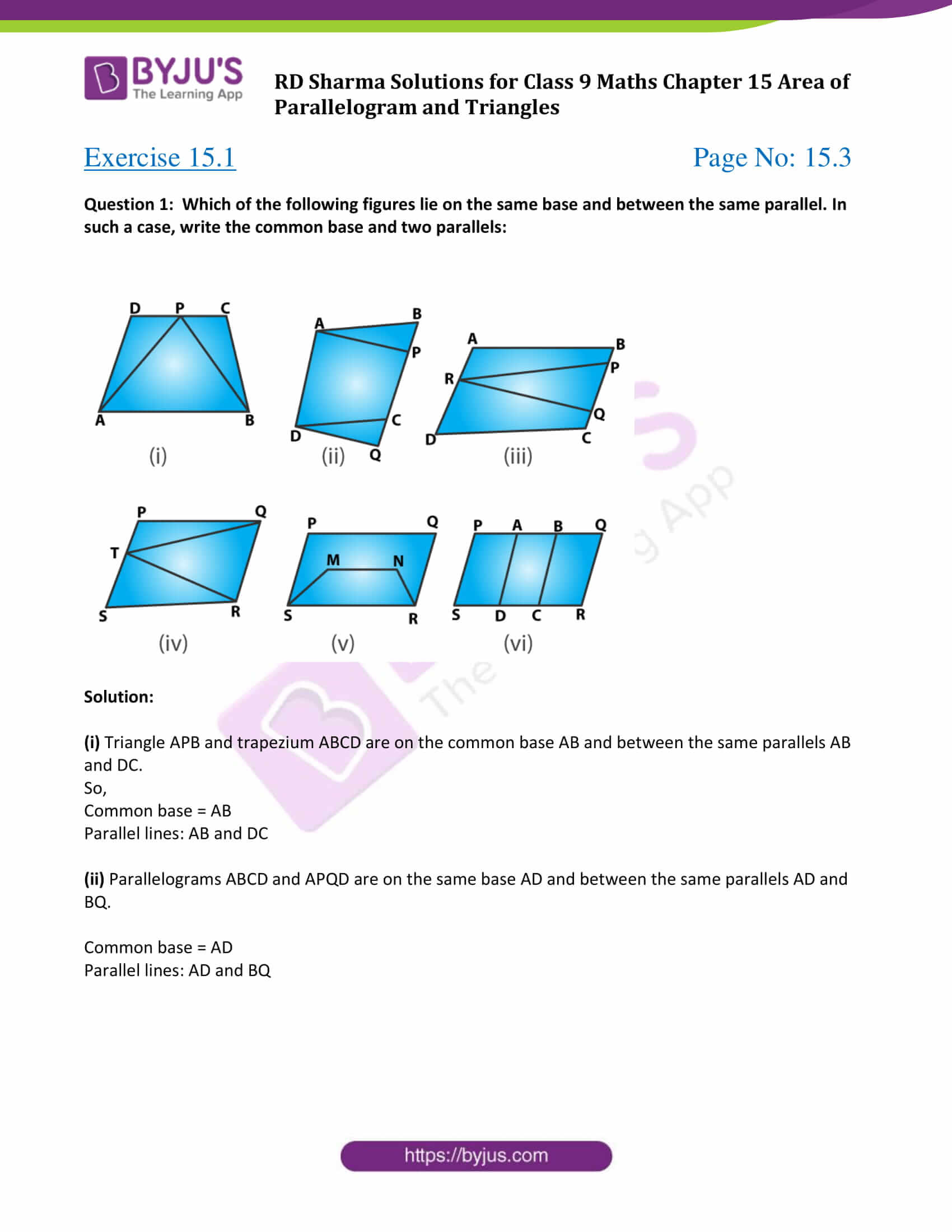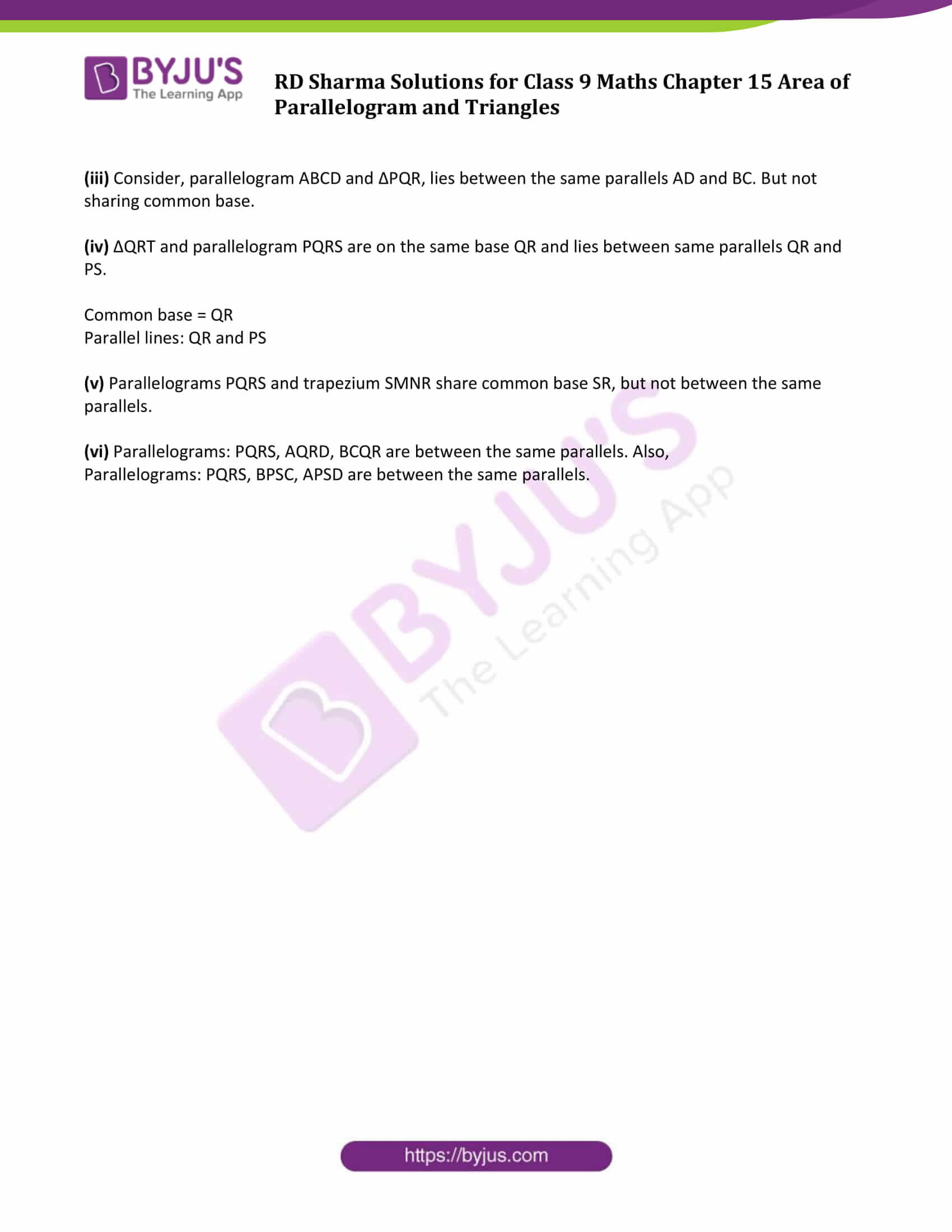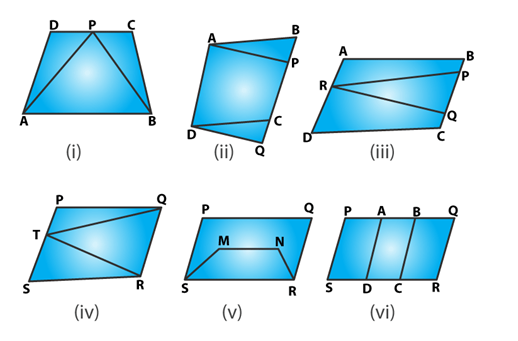# RD Sharma Solutions for Class 9 Maths Chapter 15 Area of Parallelograms and Triangles Exercise 15.1

Get free RD Sharma Solutions for Class 9 Maths Chapter 15 exercise 15.1 Area of Parallelograms and Triangles here. Exercise 15.1 of Class 9 Maths mainly deals with problem based figures on the same base and between the same parallels. Students can download the below pdf and get answers to all the questions listed in RD Sharma class 9 exercise 15.1. The RD Sharma solutions for Class 9 can help students discover new ways to solve difficult problems.

## Download PDF of RD Sharma Solutions for Class 9 Maths Chapter 15 Area of Parallelograms and Triangles Exercise 15.1### Access Answers to Maths RD Sharma Solutions for Class 9 Chapter 15 Area of Parallelograms and Triangles Exercise 15.1 Page number 15.3

Question 1: Which of the following figures lie on the same base and between the same parallel. In such a case, write the common base and two parallels:Solution:

(i) Triangle APB and trapezium ABCD are on the common base AB and between the same parallels AB and DC.

So,

Common base = AB

Parallel lines: AB and DC

(ii) Parallelograms ABCD and APQD are on the same base AD and between the same parallels AD and BQ.

(iii) Consider, parallelogram ABCD and ΔPQR, lies between the same parallels AD and BC. But not sharing common base.

(iv) ΔQRT and parallelogram PQRS are on the same base QR and lies between same parallels QR and PS.

Common base = QR

Parallel lines: QR and PS

(v) Parallelograms PQRS and trapezium SMNR share common base SR, but not between the same parallels.

(vi) Parallelograms: PQRS, AQRD, BCQR are between the same parallels. Also,

Parallelograms: PQRS, BPSC, APSD are between the same parallels.

## RD Sharma Solutions for Class 9 Maths Chapter 15 Area of Parallelograms and Triangles Exercise 15.1

RD Sharma Solutions Class 9 Maths Chapter 15 Area of Parallelograms and Triangles Exercise 15.1 is based on topic- Figures on the same base and between the same parallels. This concept helps students to identify figures lie on the same base and between the same parallel, which will be helpful further to solve complex problems.